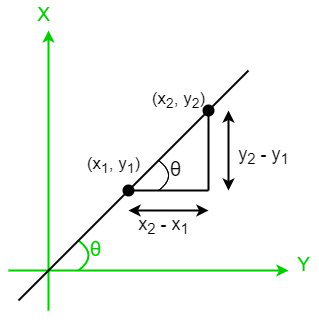# Slope Formula

• Last Updated : 15 May, 2022

A slope formula is used to determine the steepness or inclination of a line. The x and y coordinates of the points lying on the line are used to calculate the slope of a line. The change in the “y” coordinate with respect to the change in the “x” coordinates is called the slope of a line, and it is usually depicted by the letter “m”. Using the slope formula, we can determine whether two lines are perpendicular, parallel, or collinear.

### Slope Formula

In mathematics, the slope of a line is used to determine how much the line has inclined, i.e., the steepness of a line. To determine the slope of a line, we need the x and y coordinates of the points lying on the line. The slope formula is the net change in the “y” coordinate divided by the net change in the “x” coordinate. Δy is the change in the “y” coordinates, and Δx is the change in the “x” coordinates. Hence, the ratio of the change in “y” coordinates with respect to the change in the “x” coordinates is given by,

Slope (m) = change in y/change in x = Δy/Δx

m= (y2 – y1)/(x2 – x1)

Where x1 and x2 are the coordinates of the X-axis and y1 and y2 are the coordinates of the Y-axis.• We know that tan θ is also a slope of the line hence the slope of a line can also be represented as,

Slope (m) = tan θ = Δy/Δx

Where θ is the angle made by the line w.r.t to the positive X-axis,

Δy = change in the “y” coordinates,

Δx = change in the “x” coordinates.

• We can also define the slope of a line as the ratio of rising with respect to run.

Slope (m) = Rise/Run

• Let ax + by + c = 0 be the general equation of a line. Now, the formula for the slope of the line is given by,

Slope (m) = – coefficient of x/coefficient of y = -a/b

• The slope-intercept form of a line using the line equation is given as,

y = mx + c

Where m is the slope of the line and c is the y-intercept of the line.

### Sample Problems

Problem 1: Find the slope of a line whose coordinates are (3, 7) and (5, 8).

Solution:

Given, (x1, y1) = (3,7) and (x2, y2) = (5,8)

Slope formula (m) = (y2 – y1)/(x2 – x1)

⇒ m = (8 – 7)/(5 – 3) = 1/2

Hence, the slope of the given line is 1/2.

Problem 2: Determine the slope of a line whose coordinates are (7, -5) and (2, -3).

Solution:

Given, (x1, y1) = (7, -5) and (x2, y2) = (2, -3)

Slope formula (m) = (y2 – y1)/(x2 – x1)

⇒ m = (-3 – (-5))/(2 – 7) = -2/5

Hence, the slope of the given line is -2/5

Problem 3: Find the value of a, if the slope of a line passing through the points (-4, a) and (2, 5) is 3.

Solution:

Given, (x1, y1) = (4,a) and (x2, y2) = (2, 5) and slope (m) = 3

We know that slope (m) = (y2 – y1)/(x2 – x1)

⇒ 3 = (5 – a)/(2 – 4)

⇒ 3 = (5 – a)/(-2)

⇒ -6 = 5 – a ⇒ a = 5 + 6 = 11

Hence, the value of a = 11

Problem 4: If a line makes an angle of 60° with the positive Y-axis, then what is the value of the slope of the line?

Solution:

Given data, Angle made by a line with the positive y-axis = 60°

We know that if the line makes an angle of 60° from the positive y-axis, then it makes an angle of (90° – 60° = 30°) with the x-axis.

Therefore, the value of the slope of the line (m) = tan 30° = 1/√3

Hence, the value of the slope of the line = 1/√3.

Problem 5: Sheela was checking a graph, she noticed that the raise was 12 units and the run was 4 units. Now calculate the slope of a line?

Solution:

Given data, rise = 12 units and run = 4 units

We know that slope (m) = rise/run

⇒ m = 12/4 = 3

Hence, the slope of the given line is 3

Problem 6: Find the slope of the line 3x – 7y + 8 = 0.

Solution:

Given data, The equation of the line = 3x – 7y + 8 = 0

Now, compare the given with the general equation of the line i.e., ax + by + c = 0

Therefore, a = 3, b = -7 and c = 8

We know that Slope (m) = – coefficient of x/coefficient of y = -a/b

⇒ m = -3/(-7) = 3/7

Hence, the slope of the given line is 3/7.

My Personal Notes arrow_drop_up